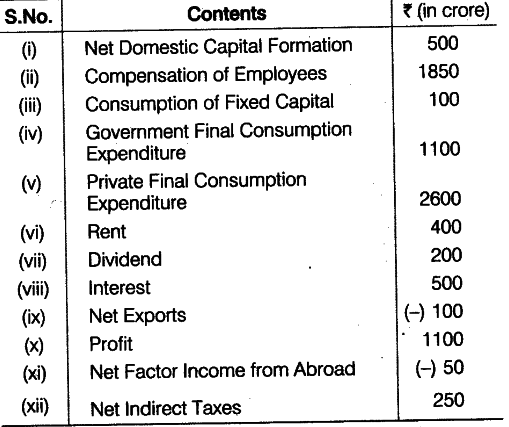# Calculate Gross National Product at Factor Cost

Calculate Gross National Product at Factor Cost by
(a) income method
(b) Expenditure method from the following data:(a) By income method,
\$GN{{P}{FC}}\$ = Compensation of Employees + (Rent+Interest+Profits)+ Net Factor Income from Abroad + Consumption of Fixed Capital =1850+(400+500+1100)+(-50)+100 = Rs. 3900 crore
(b) By expenditure method,
\$GN{{P}
{FC}}\$ = Private Final Consumption Expenditure + Government Final Consumption Expenditure + Net Domestic Capital Formation + Consumption of Fixed Capital + Net Export +Net Factor Income from Abroad - Net Indirect Taxes
= 2600 +1100 +500 +100+ (-100)+ (-50)-250 = 4300 - 400 = Rs. 3900 crore